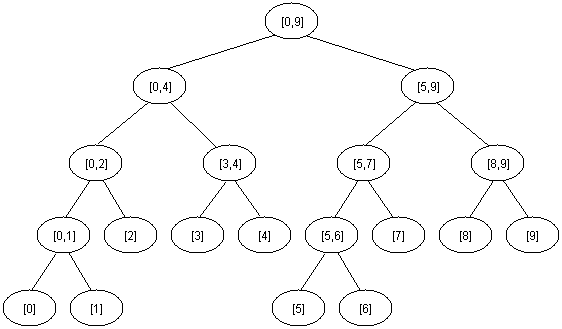### Segment Trees, Tutorial, C++ Code and Applications

A segment tree is a heap-like data structure that can be used for making update/query operations upon array intervals in logarithmical time. We define the segment tree for the interval [i, j] in the following recursive manner:
• the first node will hold the information for the interval [i, j]
• if i<j the left and right son will hold the information for the intervals [i, (i+j)/2] and [(i+j)/2+1, j]
Notice that the height of a segment tree for an interval with N elements is [logN] + 1. Here is how a segment tree for the interval [0, 9] would look like:Code for creating a segment tree recursively:
1. #include <iostream>
2. #include <stdio.h>
3. #include <stdlib.h>
4. using namespace std;
5. typedef struct segmentTreeNode* Node;
6. struct segmentTreeNode {
7. int low;
8. int high;
9. Node left;
10. Node right;
11. } segmentTreeNode;
12. Node createSegmentTree(int low, int high);
13. int main() {
14.         int N = 9;
15. Node root=createSegmentTree(0,N-1);
16. printf("%d %d",N, root->right->low);
17. return 0;
18. }

19. Node createSegmentTree(int low, int high) {
20. Node root=(Node)malloc(sizeof(segmentTreeNode));
21. root->low = low;
22. root->high = high;
23. root->left = 0;
24. root->right = 0;
25. if(low != high) {
26. Node left = createSegmentTree(low, (low+high)/2);
27. Node right = createSegmentTree(((low+high)/2)+1,high);
28. root->left=left;
29. root->right=right;
30. }
31. return root;
32. }

Applications: Range Minimum Query (RMQ) problems , Least Common Ancestor (LCA) problems etc.

For clarifications/suggesions, the comments section is all yours :)